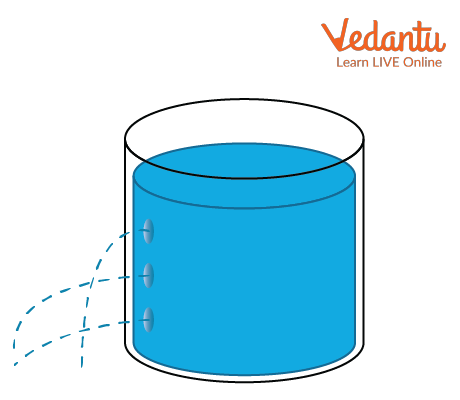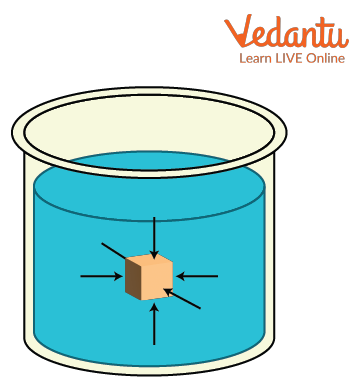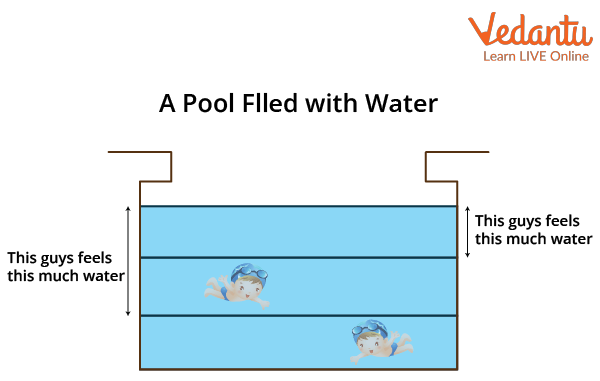Courses
Courses for Kids
Free study material
Free LIVE classes
More

# Fluid Pressure - JEE Important Topic## Fluid Pressure and Its Properties

Last updated date: 18th Mar 2023
Total views: 80.4k
Views today: 0.59k

The pressure at a point inside the fluid due to the fluid’s weight is referred to as liquid pressure or pressure in a fluid. This pressure might increase with a change in the fluid’s velocity or any hydraulic mechanism. Fluid pressure can also be defined as the force applied per unit area acting on an object placed inside a fluid or on the surface of a container.  The pressure applied by a fluid or a liquid is in all directions as liquids do not have a definite shape.  Let us discuss a few more concepts of liquid pressure.

## What is Meant by Fluid?

Anything that flows and easily deforms its shape under the influence of stress or an external force are termed as fluids. Therefore, fluids do not have a definite shape and take the shape of the container that they are placed in. Liquids and gases in general are termed as fluids. These can be classified into different types like compressible fluid, real fluid, ideal fluid, newtonian fluid, non-newtonian fluid, and so on.

## What is Fluid Pressure Or What is Liquid Pressure?

Being a scalar quantity the fluid or liquid pressure is the force per unit area wherein the force is acting in a direction perpendicular to the surface. Fluid pressure is an important concept of fluid mechanics. Additionally, it must be noted that the fluid pressure increases with depth. Let us understand fluid pressure with a few examples:

• Fluid Filled in a ContainerFluid Pressure or Liquid Pressure Increasing with Depth

The image above shows a container filled with fluid which is punctured with holes at three levels. The fluid oozes out of the top hole with the least pressure as it covers less area, whereas the fluid from the last hole oozes with the maximum pressure due to the pressure applied by the fluid above it.

• An Object Placed inside a Container filled with a Fluid or Liquid:Pressure on a Object Inside a Liquid Filled Container

At a given depth the pressure in a fluid is the same in all directions. Refer to the image below that depicts an object placed at a certain depth of a container filled with a fluid. As at a given depth pressure is the same in all directions therefore the molecules of fluid or liquid direct an equal pressure on the object. If the pressure were not to be equal the liquid or fluid would have flowed.

• A Pool Filled with WaterFluid Pressure or Liquid Pressure Increasing with Depth

A man in the pool experiences different liquid/fluid pressure at different points due to the pressure of the fluid acting above it. At the depth of the pool the man experiences the highest pressure.

• Pressure in Liquids Formula Or Pressure Formula for Fluid

Pressure as we know is denoted as $Pressure~(P)=\dfrac{Force~(F)}{Area~(A)}$

Where F is a constant force being applied on a surface area A.

Pressure is measured in Pascals (Pa) whereas Force and Area are measured in Newton (N) and m2. Thus, 1 Pa can be defined as 1 $\dfrac{N}{ m^2}$.

Pressure in a liquid’s formula is denoted as $P_{l i q}=P+\rho g h$, where P is the reference point pressure, P is the pressure at the point in a liquid, $\rho$ is the fluid density, g is acceleration due to gravity ($= 9.8\dfrac{m}{s^2}$) and h is the height from the reference point.

$\rho$ is the fluid density which can be calculated as the ratio of mass of fluid to volume of the fluid. It can be denoted as $\rho=\dfrac{m}{V}$

Substituting the same above, we get $P_{l i q}=P+ \dfrac{m}{V} gh$

Thus, the pressure formula for fluid can be calculated as $P_{l i q}=P+\rho g h$ or $P_{l i q}=P+\dfrac{m}{V} g h$

When the fluid is subjected to an atmospheric pressure the pressure of the liquid's formula is denoted as $P_{l i q}=P_0+\rho g h$, where $P_0$ is the atmospheric pressure.

## Solved Examples to Find Liquid Pressure

Example 1: If the density of gold is 19320 Kgm-3. Calculate the relative density of gold. (Given Density of water = 1000 kg m-3).

Solution:

$\text{Relative density of gold} =\dfrac{\text { density of gold }}{\text { density of water }}$

\begin{align} &\Rightarrow \text{Relative density of gold}= \dfrac{19320 \mathrm{~kg} \cdot \mathrm{m}^{3}}{1000 \mathrm{~kg} \cdot \mathrm{m}^{3}} \\ & \therefore \text{Relative density of gold}=19.32 \end{align}

Hence, the relative density of gold is 19.32.

Example 2: Calculate the pressure exerted on a scuba driver which is at 14 metres below the surface of the ocean. Assume standard atmospheric pressure.

Solution:

As we know from above that, $P_{l i q}=P_0+\rho g h$, thus P0 is the atmospheric pressure (1.01 $\times$ 105 $\dfrac{N}{m^2}$) , $\rho=$1.03 $\times$ 103 $\dfrac{kg}{m^3}$, g= 9.8 $\dfrac{m}{s^2}$ and h= 14 metres

\begin{align} &P_{l i q}=1.01 \times 10^{5}+1.03 \times 10^{3} \times 9.8 \times 14 \\ & \therefore P_{l i q}=2.42 \times 10^{2} \mathrm{kPa} \end{align}

Hence, the pressure exerted on a scuba driver which is 14 metres below the surface of the ocean is $2.42 \times 10^{2} \mathrm{kPa}$.

## Characteristics of Liquid Pressure

Some of the properties of liquid pressure include:

• Liquid Pressure increases with depth. It means that the deeper the liquidor fluid the higher is its pressure.

• Fluid pressure/liquid pressure does not depend on the shape of the container, its size and its area of surface.

• Fluid or liquid pressure is always the same at a certain level.

• At any point in a liquid the pressure acts in all directions.

• Fluid pressure acts irrespective of the surface area of the object submerged or placed in it.

## Method to Find the Centre of Pressure

The method to find the centre of pressure requires one to have a knowledge of calculus and the measure of the pressure around an object. Now, the centre of pressure is nothing but the point where the total pressure acts. It is different depending on how an object is immersed in the fluid i.e horizontally, vertically, inclined etc. The centre of pressure will be different in each case.

Or

The centre of pressure can be said as the point where the force acting on the surface of an object produces the same effect as the continuous distribution of forces on the surface in the liquid.

## Conclusion

Fluid pressure is due to the weight of the fluid which might change due to fluid velocity or any hydraulic mechanism that causes a change in fluid motion. Based on whether the fluid is in motion or not the liquid pressure is further divided into static and dynamic pressure. Fluid pressure also has certain characteristics that have to be considered while calculating the liquid pressure under different scenarios. The article presents a thorough understanding of the properties of liquid pressure and how it is measured.

## FAQs on Fluid Pressure - JEE Important Topic

1. Which device is used to measure pressure in a liquid /fluid?

The device used to measure the liquid pressure or fluid pressure is a manometer which works on  the principle of hydrostatic equilibrium. Hydrostatic equilibrium is nothing but the measure of excess pressure at a point which is equal to the weight of the liquid column above that point.  Manometer is used to measure moderate fluid pressure which consists of a tube whose one end is exposed to the atmosphere. Manometers are connected to the point where the fluid pressure is to be calculated.

2. What are the types of Fluid pressure?

The different types of fluid pressure are static pressure and dynamic pressure. Static pressure of a fluid is the one which is the same in all directions, whereas dynamic pressure is for a moving fluid or liquid. Interestingly, it also depends on the direction of motion.  As in static pressure the fluid is not moving thus, the pressure acts due to the fluid weight. It can also be said that static pressure is due to the weight of gravity acting on the molecules of the fluid. As dynamic pressure is for a moving fluid thus it can be denoted as kinetic energy per unit volume of a fluid.